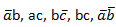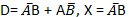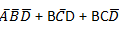# The circuit shown below is driven by a sinusoidal input vi=Vp cos(t/RC). The steady state output v0 is

1.   (Vp/3) cos(t/RC)

2.   (Vp/3) sin(t/RC)

3.  (Vp/2) cos(t/RC)

4.  (Vp/2) sin(t/RC)

4

(Vp/3) cos(t/RC)

Explanation :
No Explanation available for this question

# It is desired to generate the following three Boolean functions:

1.

2.  ab +Bc

3.  BC-AC

4.  All of these

4Explanation :
No Explanation available for this question

# The circuit shown in the figure converts

1.  BCD to binary code

2.  Binary to excess

3.  Excess-3 to Gray Code

4.  Gray to Binary code

4

Gray to Binary code

Explanation :
No Explanation available for this question

# The switching circuit given in the figure can be expressed in binary logic notation as

1.  L = (A+B) (C+D) E

2.  L = AB + CD+ E

3.  L = E+ (A+B) (C+D)

4.  L =(AB+CD)E

4

L = (A+B) (C+D) E

Explanation :
No Explanation available for this question

# If negative logic is used, diode gate shown in the given figure will represent a/an

1.  OR gate

2.  AND gate

3.  NOR gate

4.  NAND gate

4

AND gate

Explanation :
No Explanation available for this question

# Which of the following is the truth table of the given logic is true

1.   X Y Z 0 0 1 0 1 1 1 0 1 1 1 1

2.   X Y Z 0 0 0 0 1 1 1 0 1 1 1 1

3.   X Y Z 0 0 1 0 1 0 1 0 0 1 1 0

4.   X Y Z 0 0 0 0 1 1 1 0 1 1 1 0

4
 X Y Z 0 0 1 0 1 1 1 0 1 1 1 1

Explanation :
No Explanation available for this question

# For a binary half-subtractor having two inputs A and b, correct set of logical expression for the outputs D(+A minimum B) and X(= borrow) are

1.

2.

3.

4.

4Explanation :
No Explanation available for this question

# The average power delivered to an impedance (4-j3)Ω by a current 5cos(100πt + 100)A is

1.   44.2 W

2.  50 W

3.  62.5 W

4.  125 W

4

50 W

Explanation :
No Explanation available for this question

# The circuit shown in the given figure is a/an

2.  Subtractor

3.  Parity generator

4.  Comparator

4

Comparator

Explanation :
No Explanation available for this question

# In a certain application four inputs A, B, C, D are fed to logic circuits producing an output which operates a relay. The relay turns on when f(A,B,C,D) = 1 for the following states of the inputs (A B C D) : 0000, 0010, 0101, 0110, 1101 and 1110, States 1000 and 1001 do not occur, and for the remaining states the relay is off. The minimized Boolean expression f is

1.

2.

3.

4.

4